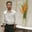Related Tags

community creator
recursion
c
c++

# How to print possible combinations of words from phone digitsBehzad Ahmad

### Problem statement

Find all the possible combinations of the words formed as a result of some number which is given by the user. The user enters a number and we have to print all the possible combinations of words that can be formed in the old mobile keyboard.

We have seen the QWERTY keypad, but before the QWERTY keypad was invented we used a keypad that consisted of 12 buttons and each button had some words and numbers associated with it.

Here is the sample keyboard:

 1 2ABC 3DEF 4GHI 5JKL 6MNO 7PQRS 8TUV 9WXYZ * 0 #

If we want to write Z we have to click the button 9 around 4 times in order to write Z.

Each button has its own associated characters and we have to use these while typing. We have a maximum of 4 options in the case of 7 and 9. Otherwise, we have 3 options.

Let’s have a look at the example to understand how it works.

If we have the input value set as 234 then the possible words formed by using this number are:

adg adh adi aeg aeh aei afg afh afi bdg bdh bdi beg beh bei bfg bfh bfi cdg cdh cdi ceg ceh cei cfg cfh cfi

We’ll implement the code of the above example with the help of recursion.

### Code

#include <iostream>
using namespace std;

char keypad[] = {"", "", "ABC", "DEF", "GHI", "JKL", "MNO", "PQRS", "TUV", "WXYZ"};

void generate(char *input, char *out, int i, int j) {
if(input[i] == '\0') {
out[j] = '\0';
cout << out << endl;
return;
}

int digit = input[i]-'0';
if(digit == 1 || digit == 0) {
generate(input, out, i + 1, j);
}

for(int k = 0; keypad[digit][k] != '\0'; k++) {
generate(input, out, i + 1, j + 1);
}
}

int main() {
char input;

cout << "Please Enter 2 to 3 Integer Numbers : ";
cin >> input;

char out;
generate(input, out, 0, 0);
return 0;
}

Enter the input below

Print words from phone digits

### Explanation

• Line 4: We specify the basic structure of the old keypad.

• Line 6: The input is basically the input words which the user has given and out stands for the output array, while i denotes the input index and j denotes output index.

• Line 7–10: It is the base case that checks if the input array reaches the last position. This means that the code is working and now we just have to print the output and terminate the program.

• Line 13–15: A recursive case in which we extract the first digit and iterate the string of the keypad array.

• Line 18–20: We recursively call for to fill out the remaining words.

• Line 24–32: We create a driver program that takes input from the user and calls the function.

RELATED TAGS

community creator
recursion
c
c++

CONTRIBUTORBehzad Ahmad
RELATED COURSES

View all Courses

Keep Exploring

Learn in-demand tech skills in half the time